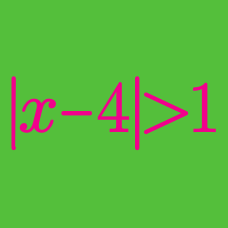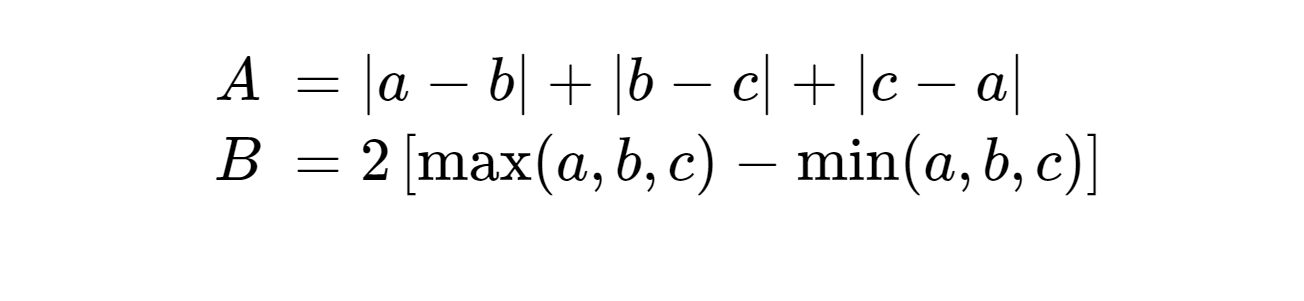Algebra

# Absolute Value Inequalities - Problem SolvingFor all ordered triples of real values $$(a, b, c)$$, which of these numbers is larger?

If $$x, y, z$$ are non-zero real, what is the range of

$f(x, y, z) = \frac{ |x+y| } { |x| + |y| } + \frac{ |y+z| } { |y| + |z| } + \frac{ |z+x| } { |z| + |x| } ?$

Consider all monic polynomials $$f(x) = x^2 + bx + c$$, where $$b$$ and $$c$$ are real numbers. What is the minimum value of $$N$$, where

$N = \max_{x \in [-22, 50]} \vert f(x) \vert?$

Consider all monic polynomials of the form $$f_a(x) = x + a$$. As $$a$$ ranges over all real numbers, what is the minimum value of $$N_a$$, where

$N_a = \max_{x \in [-13, 39] } \lvert f_a(x) \rvert ?$

If the solution to the inequality $$\lvert x+ a \rvert \leq 8$$ is $$7 \leq x \leq 23,$$ what is $$a?$$

×

Problem Loading...

Note Loading...

Set Loading...